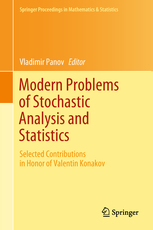• A
• A
• A
• ABC
• ABC
• ABC
• А
• А
• А
• А
• А
Regular version of the site

Regression-Based Variance Reduction Approach for Strong Approximation Schemes

P. 131-178.
Belomestny D., Häfner S., Urusov M.

In this paper we present a novel approach towards variance reduction for discretised diffusion processes. The proposed approach involves specially constructed control variates and allows for a significant reduction in the variance for the terminal functionals. In this way the complexity order of the standard Monte Carlo algorithm (ε3) can be reduced down to ε2 log(ε1) in case of the Euler scheme with ε being the precision to be achieved. These theoretical results are illustrated by several numerical examples.

In bookHeidelberg: Springer, 2017.